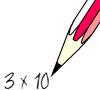# Standard FormIn science lessons, you have no doubt used scientific notation, or standard form, to write numerical answers to questions that are either very large or very small.

There are many confusions that arise when using this technique, so let's sort them out by beginning with the number 420.

In standard form we re-write it in terms of powers of ten:
420 = 4.2 × 10²

One important rule is that the number we are multiplying by ten must itself lie between 1 and 10:

NumberCorrect Standard FormIncorrect
54325·432 × 10354·32 × 102
14,5001·45 × 10414·5 × 103
N.B. Values are however numerically correct!Maths MenuGo to next page